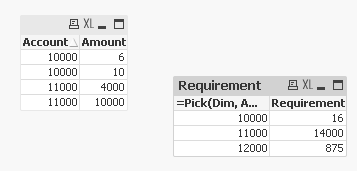# QlikView App Development

Discussion Board for collaboration related to QlikView App Development.

New Contributor III

## Ratios Based on a Dimension?

Hi all,

I'm having some trouble calculating a ratio and was wondering if anyone had run into a similar issue. I've got financial (margin, revenue, etc) and operational (headcount, etc.) data that come into my data set under specific account codes. I need to generate ratios using these numbers. The problem I'm running into is that I need to use set analysis to generate the numerator and denominator, and then assign that number to a new account code. As a result, I get null values for my new ratio.

To illustrate, my data comes in like so:

Facts:

 Account Amount 10000 10 11000 10000 10000 6 11000 4000

Dimension:

In the example above, I'd like to the result of (14000/16) to be my result for account 12000. Is this possible to do using my current approach, or do I need to modify my dimension table? Thanks.

EDIT:

I should add that my formula for the account above looks something like:

If([Account]=‘12000’

, SUM({<[Account]={‘10000’)>} Amount) / SUM({<[Account]={‘11000’)>} Amount)

, SUM(Amount))

Tags (3)
1 Solution

Accepted SolutionsMVP & Luminary

## Re: Ratios Based on a Dimension?

HI,

Try like this in attached file.

Regards,

jagan.

6 RepliesMVP

## Re: Ratios Based on a Dimension?

Something like the attachedNew Contributor III

## Re: Ratios Based on a Dimension?

Yes, this solves the issue. Thank you for the quick response!

One other question, do I need to remove the 12000 account as a dimension? I noticed in playing around with the file that if you add additional dimensions (cost center, etc.), those dimensions aren't tied to the account generated by the 'Pick' function. Thanks.MVP & Luminary

## Re: Ratios Based on a Dimension?

HI,

Try like this in attached file.

Regards,

jagan.

New Contributor III

## Re: Ratios Based on a Dimension?

That's what I was looking for. Thanks!

New Contributor III

## Re: Ratios Based on a Dimension?

I keep running into issues with this problem.

Now, I'd like to pull the expression that's aligned with from a field in the data. I've got the formulas loaded, along with the 'Rank' field that allows me to tie the formula to a specific account. After that, I keep running into a wall trying to use either the Pick function or a variable created in the load script to generate my formula. I've been relying on the following threads for guidance:

Evaluate field values as chart expressions

Interpret field values as formulas

Using formulas from excel in the straight table

As always, thanks for any help in advance.MVP

## Re: Ratios Based on a Dimension?

I think you were missing TOTAL from your expression:

Concatenate(Table)

*

INLINE [

Account, Formula, Rank

12000, SUM(TOTAL {<Account={'10000'}>}Amount) / SUM(TOTAL{<Account={'11000'}>}Amount), 1

12001, SUM(TOTAL{<Account={'11000'}>}Amount) / SUM(TOTAL{<Account={'10000'}>}Amount), 2

];

Once you make that fix, your expression seems to be working

Pick(Rank,

Num(\$(=FieldValue('Formula',1)), '##.######'),

Num(\$(=FieldValue('Formula',2)), '##.'))